# 3D Trigonometry Worksheet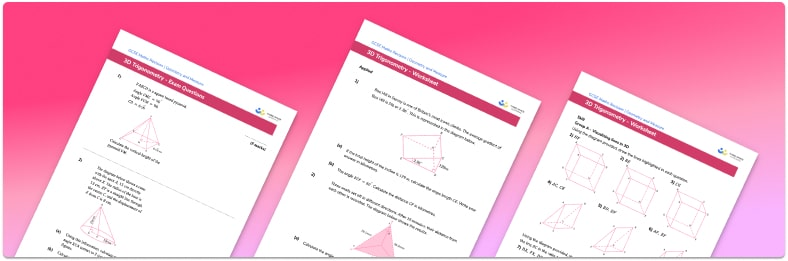Help your students prepare for their Maths GCSE with this free 3D trigonometry worksheet of 35 questions and answers

• Section 1 of the 3D trigonometry worksheet contains 27 skills-based 3D trigonometry questions, in 3 groups to support differentiation
• Section 2 contains 5 applied 3D trigonometry questions with a mix of worded problems and deeper problem solving questions
• Section 3 contains 3 foundation and higher level GCSE exam questions on 3D trigonometry
• Answers and a mark scheme for all questions are provided
• Questions follow variation theory with plenty of opportunities for students to work independently at their own level
• All questions created by fully qualified expert secondary maths teachers
• Suitable for GCSE and IGCSE maths revision for AQA, OCR and Edexcel exam boards
• To receive this resource and regular emails with more free resources, blog posts and other Third Space updates, enter your email address and click below.

• This field is for validation purposes and should be left unchanged.

You can unsubscribe at any time (each email we send will contain an easy way to unsubscribe). To find out more about how we use your data, see our privacy policy.

### 3D trigonometry at a glance

Three dimensional trigonometry involves using the trigonometry facts that we already know and applying them to 3 dimensional shapes such as cuboids and square based pyramids to work out missing angles and side lengths.

When applying trigonometry or Pythagoras’ theorem in three dimensions, we first need to create a triangle. This might require drawing an extra straight line on the shape with lengths that we know or can calculate. Sometimes we might need to use given information on the  midpoints or ratios of side lengths. Once we have a triangle, we can apply SOHCAHTOA, the sine rule or the cosine rule.

As with 2 dimensional shapes, we can decide which formula is the most appropriate by first determining whether the triangle has a right angle and then looking at what information we are given. If it is a right angle triangle then using Pythagoras’ theorem or the basic trigonometric ratios of sin, cos and tan would be the most appropriate. If it is not a right angle triangle then we need to use the sine rule or the cosine rule.

Looking forward, students can then progress to additional geometry worksheets, for example a 3D shapes worksheet or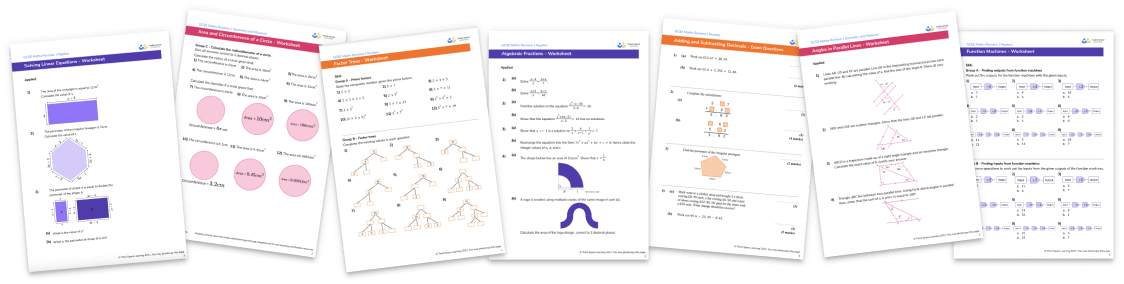For more teaching and learning support on Geometry our GCSE maths lessons provide step by step support for all GCSE maths concepts.

## Related worksheets

Exact Trig Values Worksheet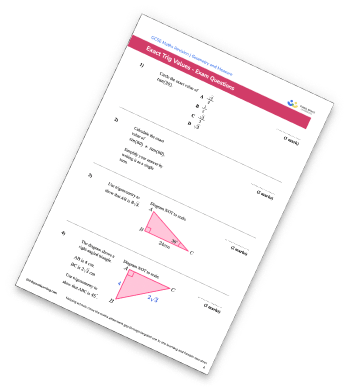Hypotenuse Worksheet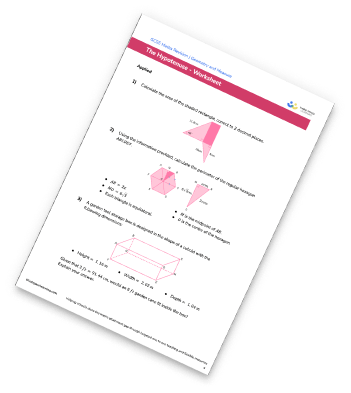Trigonometry Worksheet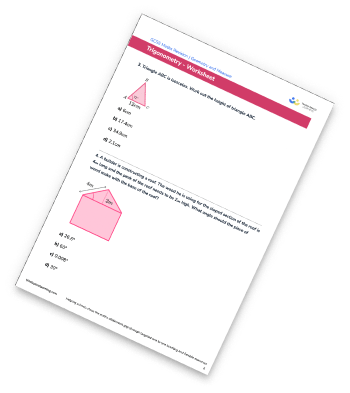Sine Rule Worksheet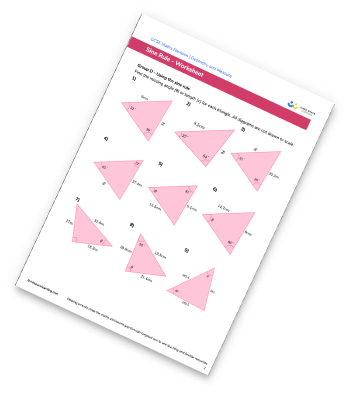## Do you have KS4 students who need more focused attention to succeed at GCSE?There will be students in your class who require individual attention to help them succeed in their maths GCSEs. In a class of 30, it’s not always easy to provide.

Help your students feel confident with exam-style questions and the strategies they’ll need to answer them correctly with our dedicated GCSE maths revision programme.

Lessons are selected to provide support where each student needs it most, and specially-trained GCSE maths tutors adapt the pitch and pace of each lesson. This ensures a personalised revision programme that raises grades and boosts confidence.

Find out more